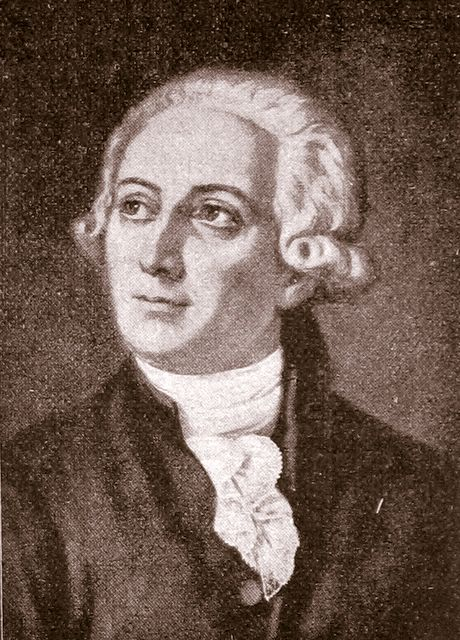## The Law of Conservation of Mass

#### Learning Objective

• Define the law of conservation of mass

#### Key Points

• The law of conservation of mass states that mass in an isolated system is neither created nor destroyed by chemical reactions or physical transformations.
• According to the law of conservation of mass, the mass of the products in a chemical reaction must equal the mass of the reactants.
• The law of conservation of mass is useful for a number of calculations and can be used to solve for unknown masses, such the amount of gas consumed or produced during a reaction.

#### Terms

• reactantAny of the participants present at the start of a chemical reaction. Also, a molecule before it undergoes a chemical change.
• law of conservation of massA law that states that mass cannot be created or destroyed; it is merely rearranged.
• productA chemical substance formed as a result of a chemical reaction.

## History of the Law of the Conservation of Mass

The ancient Greeks first proposed the idea that the total amount of matter in the universe is constant. However, Antoine Lavoisier described the law of conservation of mass (or the principle of mass/matter conservation) as a fundamental principle of physics in 1789.Antoine LavoisierA portrait of Antoine Lavoisier, the scientist credited with the discovery of the law of conservation of mass.

This law states that, despite chemical reactions or physical transformations, mass is conserved — that is, it cannot be created or destroyed — within an isolated system. In other words, in a chemical reaction, the mass of the products will always be equal to the mass of the reactants.

## The Law of Conservation of Mass-Energy

This law was later amended by Einstein in the law of conservation of mass-energy, which describes the fact that the total mass and energy in a system remain constant. This amendment incorporates the fact that mass and energy can be converted from one to another. However, the law of conservation of mass remains a useful concept in chemistry, since the energy produced or consumed in a typical chemical reaction accounts for a minute amount of mass.

We can therefore visualize chemical reactions as the rearrangement of atoms and bonds, while the number of atoms involved in a reaction remains unchanged. This assumption allows us to represent a chemical reaction as a balanced equation, in which the number of moles of any element involved is the same on both sides of the equation. An additional useful application of this law is the determination of the masses of gaseous reactants and products. If the sums of the solid or liquid reactants and products are known, any remaining mass can be assigned to gas.